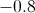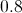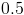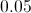# High School Math : Distributions and Curves

## Example Questions

### Example Question #1 : Understanding Normal Distributions

Which of the following populations has a precisely normal distribution?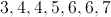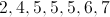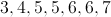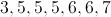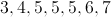Explanation:

A normal distribution is one in which the values are evenly distributed both above and below the mean.  A population has a precisely normal distribution if the mean, mode, and median are all equal.  For the population of 3,4,5,5,5,6,7, the mean, mode, and median are all 5.

### Example Question #2 : Understanding Normal Distributions

If a population has a normal distribution, the number of values within one positive standard deviation of the mean will be . . .

at least 40% of all values in the population

equal to the number of values within one negative standard deviation of the mean

fewer than the number of values within one negative standard deviation of the mean

fewer than 20% of all values in the population

equal to the number of values within two negative standard deviations of the mean

equal to the number of values within one negative standard deviation of the mean

Explanation:

In a normal distribution, the number of values within one positive standard deviation of the mean is equal to the number of values within one negative standard deviation of the mean.  The reason for this is that the values below the population mean exactly parallel the values above the mean.

### Example Question #3 : How To Find Z Scores For A Data Set

What is the-score for a value of 115 when the mean of the population is 103 and the standard deviation is 8?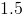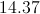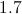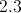Explanation:-score indicates whether a particular value is typical for a population or data set.  The closer the-score is to 0, the closer the value is to the mean of the population and the more typical it is.  The-score is calculated by subtracting the mean of a population from the particular value in question, then dividing the result by the population's standard deviation.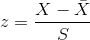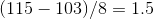### Example Question #1 : Understanding Z Scores

A value has a-score of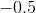.  The value is . . .

one standard deviation from the population mean

two standard deviations from the population mean

above the population mean

below the population mean

the same as the population mean

below the population mean

Explanation:

The-score indicates how close a particular value is to the population mean and whether the value is above or below the mean.  A positive-score is always above the mean and a negative-score is always below it.  Here, we know the value is below the mean because we have a negative-score.

### Example Question #1 : How To Find Z Scores For A Data Set

A population of values has a mean of 43 and a standard deviation of 12.  One of the values in the population is 49.  What is the Z-score for that value?